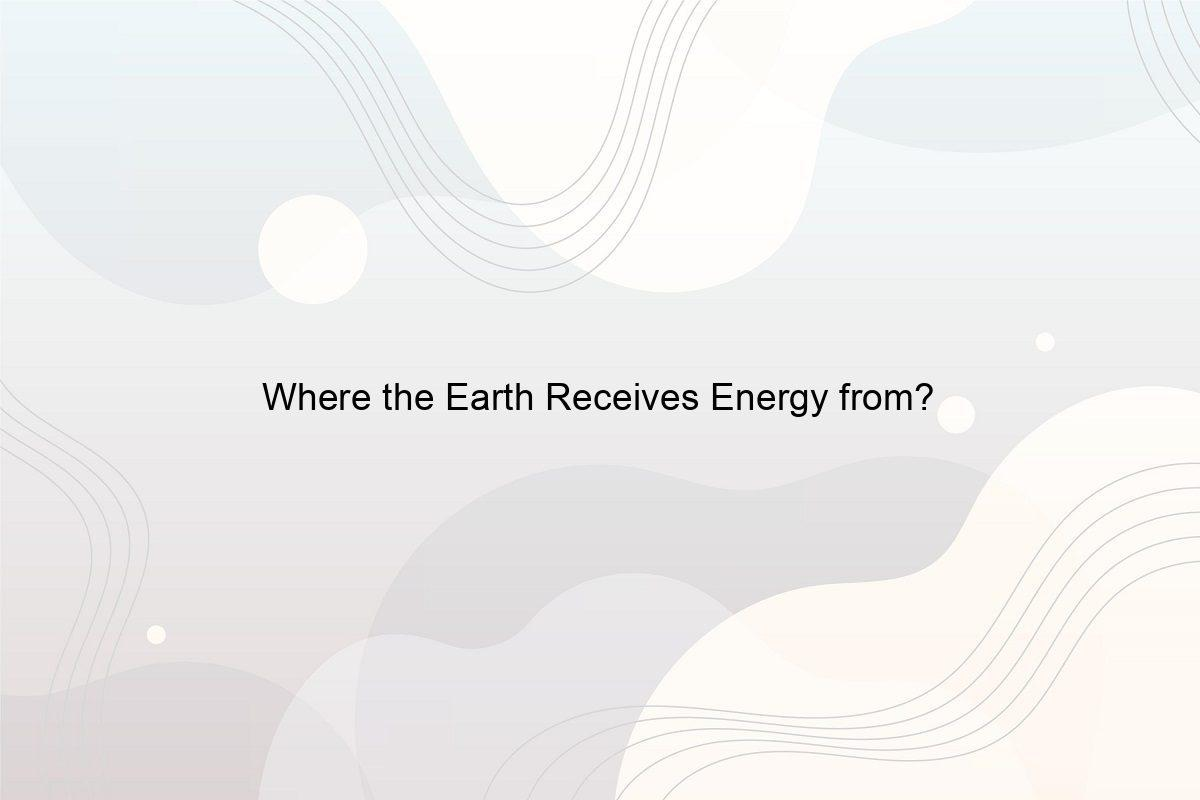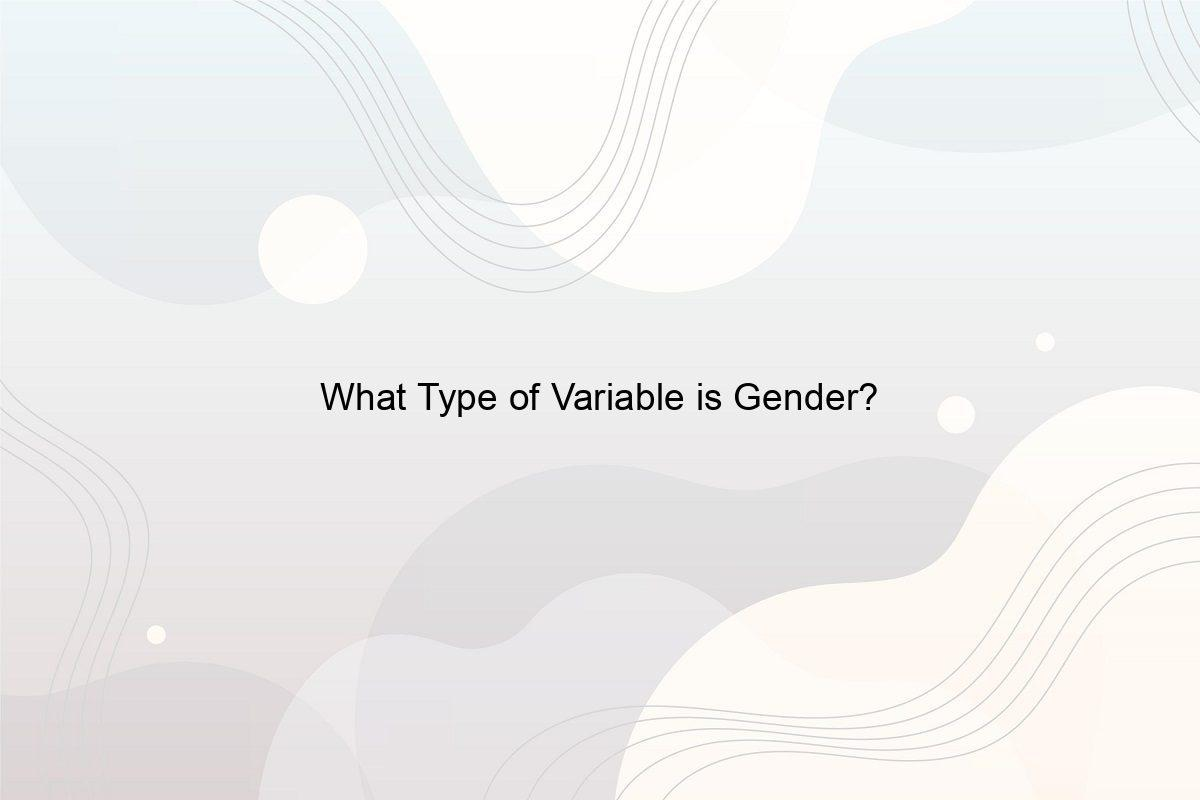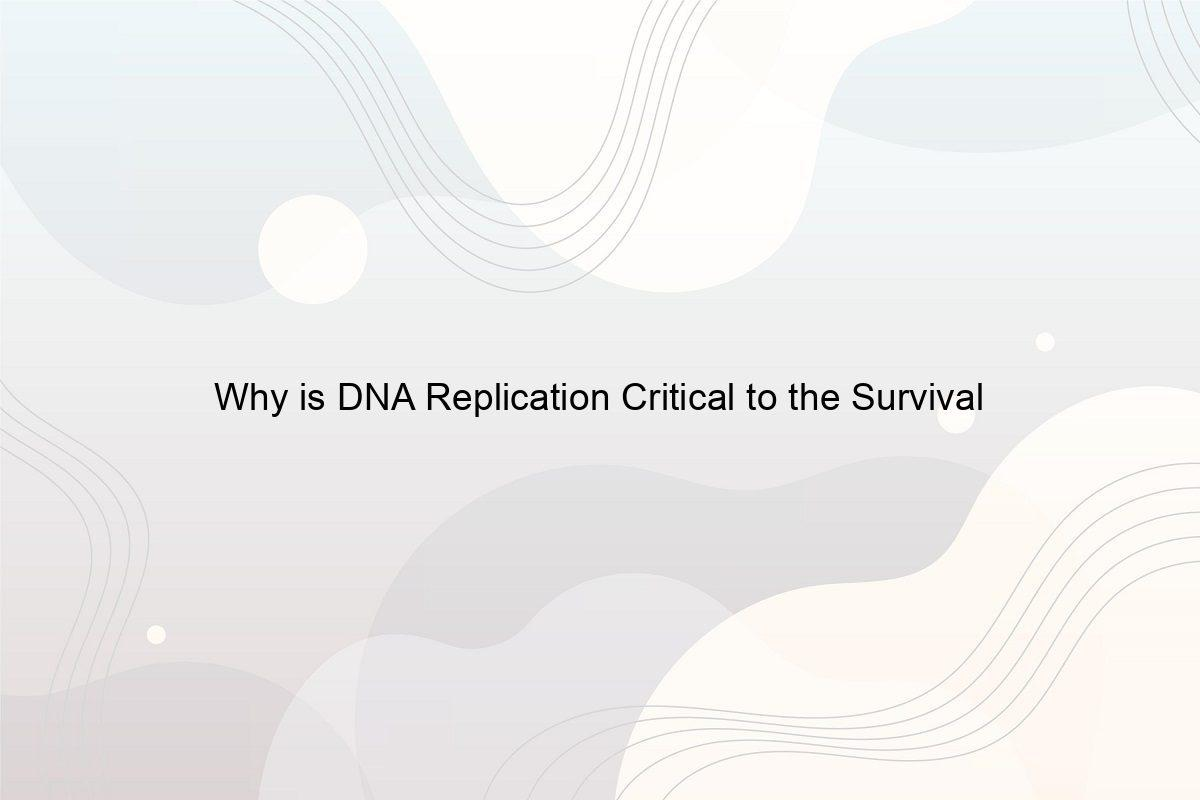﻿ What is the Potential Difference between the Ends of the Wire? - Speeli

# What is the Potential Difference between the Ends of the Wire?

How is the Current Flow determined by the Length of the Wire? How do You Calculate the Resistance and the Length? Which Device is used to Measure Resistance?

Potential difference can be defined as the voltage difference between any two points in a circuit. There is a relationship between potential difference and the length of the wire which helps the flow of current. The unit in which the potential difference is generated is called a volt. Volt (1 V) can drop over a fixed time for one-ohm resistance where the one-ampere current is flowing across the resistor. The current flows through the wires but what potential difference between the ends of the wire, it can hold up to? How do you find the potential difference at the end of a wire keeping in mind the resistance, charge, and time? Let’s begin and find it out.

### 1. How does the Potential Difference between the Ends of the Wire help Current Flow?

The potential difference in a circuit is the difference between two wires in terms of voltage. The potential difference can be written in short as p.d. It is energy that helps in moving the charged carriers between the endpoints. If the potential difference between two points 1 and 2 is ΔV = V1 − V2, then the current is flowing from point 1 towards point 2, and the charge moves in the opposite directions as V1 is greater than V2. (See What is the Difference Between Open and Closed Circuit?)

### 2. How do You Calculate the Current traveling through the Circuit?

Photo by Victor Aznabaev on Unsplash
• Learn how much is the current traveling through the circuit. These are measured in amperes.
• Check the circuit resistance which comes from a resistor. This is a device that helps in measuring the resistance from the conductor and calculating the potential difference between the ends of the wire.
• Now, multiply the total current by the amount of resistance in the circuit. The product received would be the potential difference which is measured in volts. This is called ohm’s law and is represented as V=IR.

### 3. If the Potential difference in a Circuit is Doubled what happens to the Resistance?

There is no change in the resistance of the circuit if the potential difference between the ends of the wire and the circuit current is changing with respect to each other. Resistance remains the same according to ohm’s law of voltage, V= IR. Moreover, we should not increase the voltage to a huge extent as it may destroy the whole circuit. (Also read Who Discovered Electricity?)

### 4. How do You Find the Potential Difference at the End of a Wire?

The formula to find the potential difference at the end of the wire is potential difference = work done/charge and the potential difference can only be found between two potentials only.

So, let’s find the potential difference between two points or ends of wires, 1 and 2. It will be ΔV = V1 − V2 and here V1 is greater than V2 to give it a positive value of voltage. You can find the potential at the end of the wire i.e. potential of any endpoint using this formula. Moreover, you can use the KVL voltage equation inside the circuit to calculate the potential difference between the ends of the wire, if you already know the other required values of resistors, current, etc.

### 5. How do You Find the Potential Difference when the Resistance is 3 ohm and the Current is 1.5 A?

As per ohm law, V= IR, where current I = 1.5A and resistance, R = 3 ohm.

Therefore, V= 1.5 × 3 = 4.5 V. Must read How is Energy Measured?

### 6. What is the Relationship between Potential Difference and Length of Wire in a Circuit?

The potential difference or the resistance of the circuit can be dropped to zero if the circuit wire is shorted or the resistor is shorted.

However, according to Ohm’s law, V= IR, so if we assume the current to be a constant quantity, voltage and the resistance will be directly proportional to each other, i.e. V ∝ R. And, we know from the resistance formula, resistance increase or decrease with the same respective change in the length of the wire. Hence we can establish that the potential difference and the length of the resistor wire are directly proportional to each other.

### 7. How does the Relationship between Potential Difference and Length of Wire calculated?

From Ohm’s law, V= IR, so if we assume the current flowing through the electric circuit to be a constant figure, then V ∝ R.

Moreover, from the resistance formula, the resistance can be calculated with the formula, R = ρ (l/A), or we can say,
R = [resistivity (length of the wire/ area of the wire)].

The length of the wire can be calculated with the help of this formula. Here resistance increases or decreases with the same respective change in the length of the wire, i.e. R ∝ l.

Hence from the proportional relations, V ∝ R and R ∝ l, we can establish that the potential difference and the length of the wire are directly proportional to each other (V ∝ l), i.e. can be explained as:

• If the wire length increases the resistance increases.
• If the wire length decreases the resistance also decreases.

### 8. How do You Calculate the Potential Difference between Two Points?

Photo by israel palacio on Unsplash

When you calculate the potential difference between the ends of the wire points always considers these points:

• Know the strength of the field E and the distance between the two points denoted as d.
• Use the formula ΔV = Ed to calculate the endpoints of the wire.

And the potential difference between two points or ends of wires, 1 and 2, will be ΔV = V1 − V2 where V1 > V2 to generate a positive value of voltage.

### 9. Does the Potential Difference Increase with an Increase in Electric Power?

Yes, if the potential difference of the wire is doubled, it will increase the power, assuming the resistor value remains constant. It can be solved with this formula, power, P = V²/R, where voltage and resistance are denoted as V and R respectively. Hence we can say that, P ∝ V, or directly related to each other (Also read What is a bad conductor of electricity?)

##### Related Posts## Marijuana: 8 Reasons Why was it made illegal in the first place

Why is Marijuana illegal? Why was it made illegal in the first place.## Where the Earth Receives Energy from?

What is the Primary Source of the Earth? How is the Sun's Energy received on Earth? What is Earth's Internal Heat? What is External Energy?